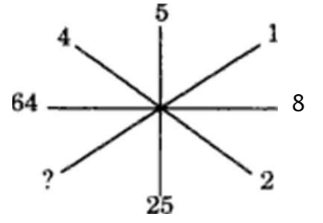#### Inserting and Missing Character

Direction: Find the missing character in each of the following questions :

1. NA1. 1
2. 2
3. 3
4. 4
##### Correct Option: A

The two ends of each line segment contain a number and its square.
We have , 22 = 4
52 = 25
82 = 64
So, missing number = 12 = ?
? = 1